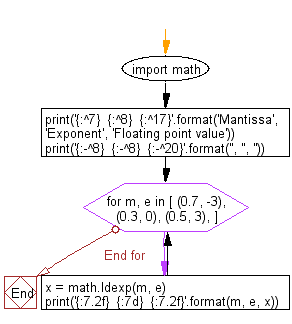﻿ Python Math: Print the floating point from mantissa, exponent pair - w3resource# Python Math: Print the floating point from mantissa, exponent pair

## Python Math: Exercise-58 with Solution

Write a Python program to print the floating point from mantissa, exponent pair.

Sample Solution:-

Python Code:

``````import math

print('{:^7}  {:^8}  {:^17}'.format('Mantissa', 'Exponent', 'Floating point value'))
print('{:-^8}  {:-^8}  {:-^20}'.format('', '', ''))

for m, e in [ (0.7, -3),
(0.3,  0),
(0.5,  3),
]:
x = math.ldexp(m, e)
print('{:7.2f}  {:7d}  {:7.2f}'.format(m, e, x))
```
```

Sample Output:

```Mantissa  Exponent  Floating point value
--------  --------  --------------------
0.70       -3     0.09
0.30        0     0.30
0.50        3     4.00
```

Flowchart:## Visualize Python code execution:

The following tool visualize what the computer is doing step-by-step as it executes the said program:

Python Code Editor:

Have another way to solve this solution? Contribute your code (and comments) through Disqus.

What is the difficulty level of this exercise?

Test your Programming skills with w3resource's quiz.

﻿

```>>> students = [{'name': 'John', 'score': 98}, {'name': 'Mike', 'score': 94}, {'name': 'Jennifer', 'score': 99}]